true# Michael Thomas## Setting the foundation right through fundamentals.

Chembur Basant Garden, Mumbai, India - 400071.

1 Student taught

Referral Discount: Get ₹ 500 off when you make a payment to start classes. Get started by Booking a Demo.

Details verified of Michael Thomas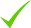IdentityEducation

Know how UrbanPro verifies Tutor details

Identity is verified based on matching the details uploaded by the Tutor with government databases.

## Overview

I have experience teaching through various classes from 5th to BTech students. I follow this profession as I enjoy teaching.Most of my teaching experience comes from voluntary activities during my college days. I have strong applied knowledge of Science and Mathematics and I try to set the foundations rights for the students for their future studies and career choices.
I am working as a Data Scientist having deep knowledge on statistics, machine learning and data science.

## Languages Spoken

Malayalam Mother Tongue (Native)

English Proficient

Tamil Basic

## Education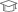Indian Insitiute Of Technology, Madras 2019

Bachelor of Technology (B.Tech.)

Chembur Basant Garden, Mumbai, India - 400071

## Verified Info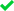ID VerifiedEducation VerifiedPhone VerifiedEmail VerifiedFacebook Verified

Report this Profile

Is this listing inaccurate or duplicate? Any other problem?

Please tell us about the problem and we will fix it.

Please describe the problem that you see in this page.

Type the letters as shown below *

Please enter the letters as show below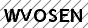## Teaches

BTech Tuition

Class LocationOnline Classes (Video Call via UrbanPro LIVE)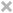Student's HomeTutor's Home

Years of Experience in BTech Tuition

2

BTech Computer Science subjects

Artificial Intelligence, Machine Intelligence, Machine Learning, Natural Language Processing, Big Data Analytics

BTech Branch

BTech Computer Science Engineering

Type of class

Regular Classes, Crash Course

Class strength catered to

One on one/ Private Tutions

Taught in School or College

No

Python Training classes

Class LocationOnline Classes (Video Call via UrbanPro LIVE)Student's HomeTutor's Home

Years of Experience in Python Training classes

2

Course Duration provided

3-6 months, 6-12 months, 1-3 months

Seeker background catered to

Individual, Educational Institution

Certification provided

No

Python applications taught

Web Scraping with Python , Data Analysis with Python , Machine Learning with Python, Automation with Python , Text Processing with Python, Help in assignment, Scipy Stack with Python , Data Science with Python, Data Extraction with Python , Regular Expressions with Python , Data Visualization with Python

Data Science Classes

Class LocationOnline Classes (Video Call via UrbanPro LIVE)Student's HomeTutor's Home

Years of Experience in Data Science Classes

2

Data science techniques

Machine learning, Python, Artificial Intelligence, R Programming

Deep Learning Training

Class LocationOnline Classes (Video Call via UrbanPro LIVE)I am Willing to TravelTutor's Home

Years of Experience in Deep Learning Training

1

Deep_Learning_Techniques

Python, Tensorflow

R Programming

Class LocationOnline Classes (Video Call via UrbanPro LIVE)Student's HomeTutor's Home

Years of Experience in R Programming

1

## Reviews

No Reviews yet! Be the first one to Review

## FAQs

1. Which BTech branches do you tutor for?

BTech Computer Science Engineering

2. Do you have any prior teaching experience?

No

3. Which classes do you teach?

I teach BTech Tuition, Data Science, Deep Learning, Python Training and R Programming Classes.

4. Do you provide a demo class?

Yes, I provide a paid demo class.

5. How many years of experience do you have?

I have been teaching for 2 years.

## Answers by Michael Thomas (8)

Answered on 02/08/2019 Learn CBSE/Class 10/Mathematics/UNIT V: Trigonometry/Introduction to Trigonometry/NCERT Solutions/Exercise 8.1

If evaluate: (ii) ...more
If evaluate:

(ii)

i) Theorems used: ...more

i)

Theorems used:

Dislike Bookmark

Answered on 02/08/2019 Learn CBSE/Class 9/Mathematics/Unit 4-Geometry/Quadrilaterals/NCERT Solutions/Exercise 8.1

Let ABCD be a parallelogram. Let P be the point where diagonal AC and BD. Let AC = BD. Then from property of parallelogram (diagonals bisect each other), AP = PC = PD = BP. Consider traingle APD. AP = DP, hence it is a isoceles triangle. Thus Angle DAP and PDA are are equal. Similarly Angle PCB and PBC... ...more

Let ABCD be a parallelogram. Let P be the point where diagonal AC and BD.

Let AC = BD. Then from property of parallelogram (diagonals bisect each other), AP = PC = PD = BP.

Consider traingle APD. AP = DP, hence it is a isoceles triangle. Thus Angle DAP and PDA are are equal.

Similarly Angle PCB and PBC are equal.

Angle APD and Angle CPB are equal (Opposite angles of intersecting lines)

Hence, Angles DAP, ADP, PCB, PBC are equal.

Similarly Angles PDC, PCD, PAB, PBA are equal.

But Angle PAB = Angle PCD. Interior alternate anlges of line interecting parallel lines.

Thus all the angles are equal and are right angle.

Rectangle is a parallelogram with all angles are right angles.

Dislike Bookmark

Answered on 02/08/2019 Learn CBSE/Class 10/Mathematics/UNIT V: Trigonometry/Introduction to Trigonometry/NCERT Solutions/Exercise 8.1

Just draw a triangle. From secant we know cosine is 12/13. Thus adjacent side is a multiple of 12 (12x)and Hypotenuse is a multiple of 13 (13x). Using pythagoras theorem, opposite side has length of 5x. Just plug these values and find them. sine = 5/13 cosine = 12/13 tangent =5/12 cotangent =12/5 cosecant... ...more

Just draw a triangle. From secant we know cosine is 12/13. Thus adjacent side is a multiple of  12 (12x)and Hypotenuse is a multiple of  13 (13x). Using pythagoras theorem, opposite side has length of 5x.

Just plug these values and find them.

sine = 5/13

cosine = 12/13

tangent =5/12

cotangent =12/5

cosecant =13/5

Dislike Bookmark

Answered on 02/08/2019 Learn CBSE/Class 9/Mathematics/Unit 4-Geometry/Quadrilaterals/NCERT Solutions/Exercise 8.1

ABCD is a rhombus. Show that diagonal AC bisects ∠ A as well as ∠ C and diagonal BDbisects ∠... ...more
ABCD is a rhombus. Show that diagonal AC bisects ∠ A as well as ∠ C and diagonal BD
bisects ∠ B as well as ∠ D.

Rhombus is a parallelogram with all sides of the same length.Just consider diagonal AC. It is intercept of two parallel lines. Thus the alternate interior sides are equal. Hence Angle CAB and Angle ACD are equal. Triangle ABC is an isosceles triangle. Thus Angle BAC and Angle BCA are equal.Combining... ...more

Rhombus is a parallelogram with all sides of the same length.
Just consider diagonal AC. It is intercept of two parallel lines. Thus the alternate interior sides are equal. Hence Angle CAB and Angle ACD are equal.
Triangle ABC is an isosceles triangle. Thus Angle BAC and Angle BCA are equal.
Combining both we get Angle ACB, and Angle ACD is equal. Thus Diagonal AC bisects Angle C.
Similar are all the rest.

Dislike Bookmark

Mitochondria
Dislike Bookmark

## Teaches

BTech Tuition

Class LocationOnline Classes (Video Call via UrbanPro LIVE)Student's HomeTutor's Home

Years of Experience in BTech Tuition

2

BTech Computer Science subjects

Artificial Intelligence, Machine Intelligence, Machine Learning, Natural Language Processing, Big Data Analytics

BTech Branch

BTech Computer Science Engineering

Type of class

Regular Classes, Crash Course

Class strength catered to

One on one/ Private Tutions

Taught in School or College

No

Python Training classes

Class LocationOnline Classes (Video Call via UrbanPro LIVE)Student's HomeTutor's Home

Years of Experience in Python Training classes

2

Course Duration provided

3-6 months, 6-12 months, 1-3 months

Seeker background catered to

Individual, Educational Institution

Certification provided

No

Python applications taught

Web Scraping with Python , Data Analysis with Python , Machine Learning with Python, Automation with Python , Text Processing with Python, Help in assignment, Scipy Stack with Python , Data Science with Python, Data Extraction with Python , Regular Expressions with Python , Data Visualization with Python

Data Science Classes

Class LocationOnline Classes (Video Call via UrbanPro LIVE)Student's HomeTutor's Home

Years of Experience in Data Science Classes

2

Data science techniques

Machine learning, Python, Artificial Intelligence, R Programming

Deep Learning Training

Class LocationOnline Classes (Video Call via UrbanPro LIVE)I am Willing to TravelTutor's Home

Years of Experience in Deep Learning Training

1

Deep_Learning_Techniques

Python, Tensorflow

R Programming

Class LocationOnline Classes (Video Call via UrbanPro LIVE)Student's HomeTutor's Home

Years of Experience in R Programming

1

No Reviews yet! Be the first one to Review

## Answers by Michael Thomas (8)

Answered on 02/08/2019 Learn CBSE/Class 10/Mathematics/UNIT V: Trigonometry/Introduction to Trigonometry/NCERT Solutions/Exercise 8.1

If evaluate: (ii) ...more
If evaluate:

(ii)

i) Theorems used: ...more

i)

Theorems used:

Dislike Bookmark

Answered on 02/08/2019 Learn CBSE/Class 9/Mathematics/Unit 4-Geometry/Quadrilaterals/NCERT Solutions/Exercise 8.1

Let ABCD be a parallelogram. Let P be the point where diagonal AC and BD. Let AC = BD. Then from property of parallelogram (diagonals bisect each other), AP = PC = PD = BP. Consider traingle APD. AP = DP, hence it is a isoceles triangle. Thus Angle DAP and PDA are are equal. Similarly Angle PCB and PBC... ...more

Let ABCD be a parallelogram. Let P be the point where diagonal AC and BD.

Let AC = BD. Then from property of parallelogram (diagonals bisect each other), AP = PC = PD = BP.

Consider traingle APD. AP = DP, hence it is a isoceles triangle. Thus Angle DAP and PDA are are equal.

Similarly Angle PCB and PBC are equal.

Angle APD and Angle CPB are equal (Opposite angles of intersecting lines)

Hence, Angles DAP, ADP, PCB, PBC are equal.

Similarly Angles PDC, PCD, PAB, PBA are equal.

But Angle PAB = Angle PCD. Interior alternate anlges of line interecting parallel lines.

Thus all the angles are equal and are right angle.

Rectangle is a parallelogram with all angles are right angles.

Dislike Bookmark

Answered on 02/08/2019 Learn CBSE/Class 10/Mathematics/UNIT V: Trigonometry/Introduction to Trigonometry/NCERT Solutions/Exercise 8.1

Just draw a triangle. From secant we know cosine is 12/13. Thus adjacent side is a multiple of 12 (12x)and Hypotenuse is a multiple of 13 (13x). Using pythagoras theorem, opposite side has length of 5x. Just plug these values and find them. sine = 5/13 cosine = 12/13 tangent =5/12 cotangent =12/5 cosecant... ...more

Just draw a triangle. From secant we know cosine is 12/13. Thus adjacent side is a multiple of  12 (12x)and Hypotenuse is a multiple of  13 (13x). Using pythagoras theorem, opposite side has length of 5x.

Just plug these values and find them.

sine = 5/13

cosine = 12/13

tangent =5/12

cotangent =12/5

cosecant =13/5

Dislike Bookmark

Answered on 02/08/2019 Learn CBSE/Class 9/Mathematics/Unit 4-Geometry/Quadrilaterals/NCERT Solutions/Exercise 8.1

ABCD is a rhombus. Show that diagonal AC bisects ∠ A as well as ∠ C and diagonal BDbisects ∠... ...more
ABCD is a rhombus. Show that diagonal AC bisects ∠ A as well as ∠ C and diagonal BD
bisects ∠ B as well as ∠ D.

Rhombus is a parallelogram with all sides of the same length.Just consider diagonal AC. It is intercept of two parallel lines. Thus the alternate interior sides are equal. Hence Angle CAB and Angle ACD are equal. Triangle ABC is an isosceles triangle. Thus Angle BAC and Angle BCA are equal.Combining... ...more

Rhombus is a parallelogram with all sides of the same length.
Just consider diagonal AC. It is intercept of two parallel lines. Thus the alternate interior sides are equal. Hence Angle CAB and Angle ACD are equal.
Triangle ABC is an isosceles triangle. Thus Angle BAC and Angle BCA are equal.
Combining both we get Angle ACB, and Angle ACD is equal. Thus Diagonal AC bisects Angle C.
Similar are all the rest.

Dislike Bookmark

Mitochondria
Dislike Bookmark

Michael Thomas describes himself as Setting the foundation right through fundamentals.. He conducts classes in BTech Tuition, Data Science and Deep Learning. Michael is located in Chembur Basant Garden, Mumbai. Michael takes at students Home and Online Classes- via online medium. He has 2 years of teaching experience . Michael has completed Bachelor of Technology (B.Tech.) from Indian Insitiute Of Technology, Madras in 2019. He is well versed in Tamil, English and Malayalam.

•• Want to learn from Michael Thomas?

• Book a Demo
X

Recommended Profiles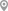Bandra Kurla Complex, MumbaiMunnekollal, BangaloreAmeerpet, HyderabadDanavaipeta, RajahmundryGadital, PuneHITEC City, Hyderabad

Reply to 's review